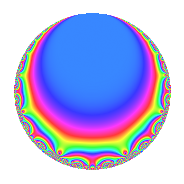# Properties

 Label 8024.2.a.rLevel 8024 Weight 2 Character orbit 8024.a Self dual Yes Analytic conductor 64.072 Analytic rank 1 Dimension 2 CM No Inner twists 1

# Related objects

## Newspace parameters

 Level: $$N$$ = $$8024 = 2^{3} \cdot 17 \cdot 59$$ Weight: $$k$$ = $$2$$ Character orbit: $$[\chi]$$ = 8024.a (trivial)

## Newform invariants

 Self dual: Yes Analytic conductor: $$64.0719625819$$ Analytic rank: $$1$$ Dimension: $$2$$ Coefficient field: $$\Q(\sqrt{5})$$ Coefficient ring: $$\Z[a_1, \ldots, a_{5}]$$ Coefficient ring index: $$2$$ Fricke sign: $$1$$ Sato-Tate group: $\mathrm{SU}(2)$

## $q$-expansion

Coefficients of the $$q$$-expansion are expressed in terms of $$\beta = \sqrt{5}$$. We also show the integral $$q$$-expansion of the trace form.

 $$f(q)$$ $$=$$ $$q + q^{3} + ( -2 - \beta ) q^{5} + \beta q^{7} -2 q^{9} +O(q^{10})$$ $$q + q^{3} + ( -2 - \beta ) q^{5} + \beta q^{7} -2 q^{9} + ( 3 - \beta ) q^{11} + ( -1 + \beta ) q^{13} + ( -2 - \beta ) q^{15} - q^{17} + ( -1 - 2 \beta ) q^{19} + \beta q^{21} + ( 7 - \beta ) q^{23} + ( 4 + 4 \beta ) q^{25} -5 q^{27} + ( -4 + 3 \beta ) q^{29} + ( 7 + \beta ) q^{31} + ( 3 - \beta ) q^{33} + ( -5 - 2 \beta ) q^{35} -8 q^{37} + ( -1 + \beta ) q^{39} + ( 5 - 2 \beta ) q^{41} + ( -3 - \beta ) q^{43} + ( 4 + 2 \beta ) q^{45} + ( 6 + 2 \beta ) q^{47} -2 q^{49} - q^{51} + ( -6 + 3 \beta ) q^{53} + ( -1 - \beta ) q^{55} + ( -1 - 2 \beta ) q^{57} - q^{59} + ( -9 - \beta ) q^{61} -2 \beta q^{63} + ( -3 - \beta ) q^{65} + ( 5 + \beta ) q^{67} + ( 7 - \beta ) q^{69} -4 q^{71} + ( 3 - 3 \beta ) q^{73} + ( 4 + 4 \beta ) q^{75} + ( -5 + 3 \beta ) q^{77} + ( 6 + \beta ) q^{79} + q^{81} + ( 1 + \beta ) q^{83} + ( 2 + \beta ) q^{85} + ( -4 + 3 \beta ) q^{87} + ( -2 + 4 \beta ) q^{89} + ( 5 - \beta ) q^{91} + ( 7 + \beta ) q^{93} + ( 12 + 5 \beta ) q^{95} -2 q^{97} + ( -6 + 2 \beta ) q^{99} +O(q^{100})$$ $$\operatorname{Tr}(f)(q)$$ $$=$$ $$2q + 2q^{3} - 4q^{5} - 4q^{9} + O(q^{10})$$ $$2q + 2q^{3} - 4q^{5} - 4q^{9} + 6q^{11} - 2q^{13} - 4q^{15} - 2q^{17} - 2q^{19} + 14q^{23} + 8q^{25} - 10q^{27} - 8q^{29} + 14q^{31} + 6q^{33} - 10q^{35} - 16q^{37} - 2q^{39} + 10q^{41} - 6q^{43} + 8q^{45} + 12q^{47} - 4q^{49} - 2q^{51} - 12q^{53} - 2q^{55} - 2q^{57} - 2q^{59} - 18q^{61} - 6q^{65} + 10q^{67} + 14q^{69} - 8q^{71} + 6q^{73} + 8q^{75} - 10q^{77} + 12q^{79} + 2q^{81} + 2q^{83} + 4q^{85} - 8q^{87} - 4q^{89} + 10q^{91} + 14q^{93} + 24q^{95} - 4q^{97} - 12q^{99} + O(q^{100})$$

## Embeddings

For each embedding $$\iota_m$$ of the coefficient field, the values $$\iota_m(a_n)$$ are shown below.

For more information on an embedded modular form you can click on its label.

Label $$\iota_m(\nu)$$ $$a_{2}$$ $$a_{3}$$ $$a_{4}$$ $$a_{5}$$ $$a_{6}$$ $$a_{7}$$ $$a_{8}$$ $$a_{9}$$ $$a_{10}$$
1.1
 1.61803 −0.618034
0 1.00000 0 −4.23607 0 2.23607 0 −2.00000 0
1.2 0 1.00000 0 0.236068 0 −2.23607 0 −2.00000 0
 $$n$$: e.g. 2-40 or 990-1000 Significant digits: Format: Complex embeddings Normalized embeddings Satake parameters Satake angles

## Inner twists

This newform does not admit any (nontrivial) inner twists.

## Atkin-Lehner signs

$$p$$ Sign
$$2$$ $$1$$
$$17$$ $$1$$
$$59$$ $$1$$

## Hecke kernels

This newform can be constructed as the intersection of the kernels of the following linear operators acting on $$S_{2}^{\mathrm{new}}(\Gamma_0(8024))$$:

 $$T_{3} - 1$$ $$T_{5}^{2} + 4 T_{5} - 1$$ $$T_{7}^{2} - 5$$